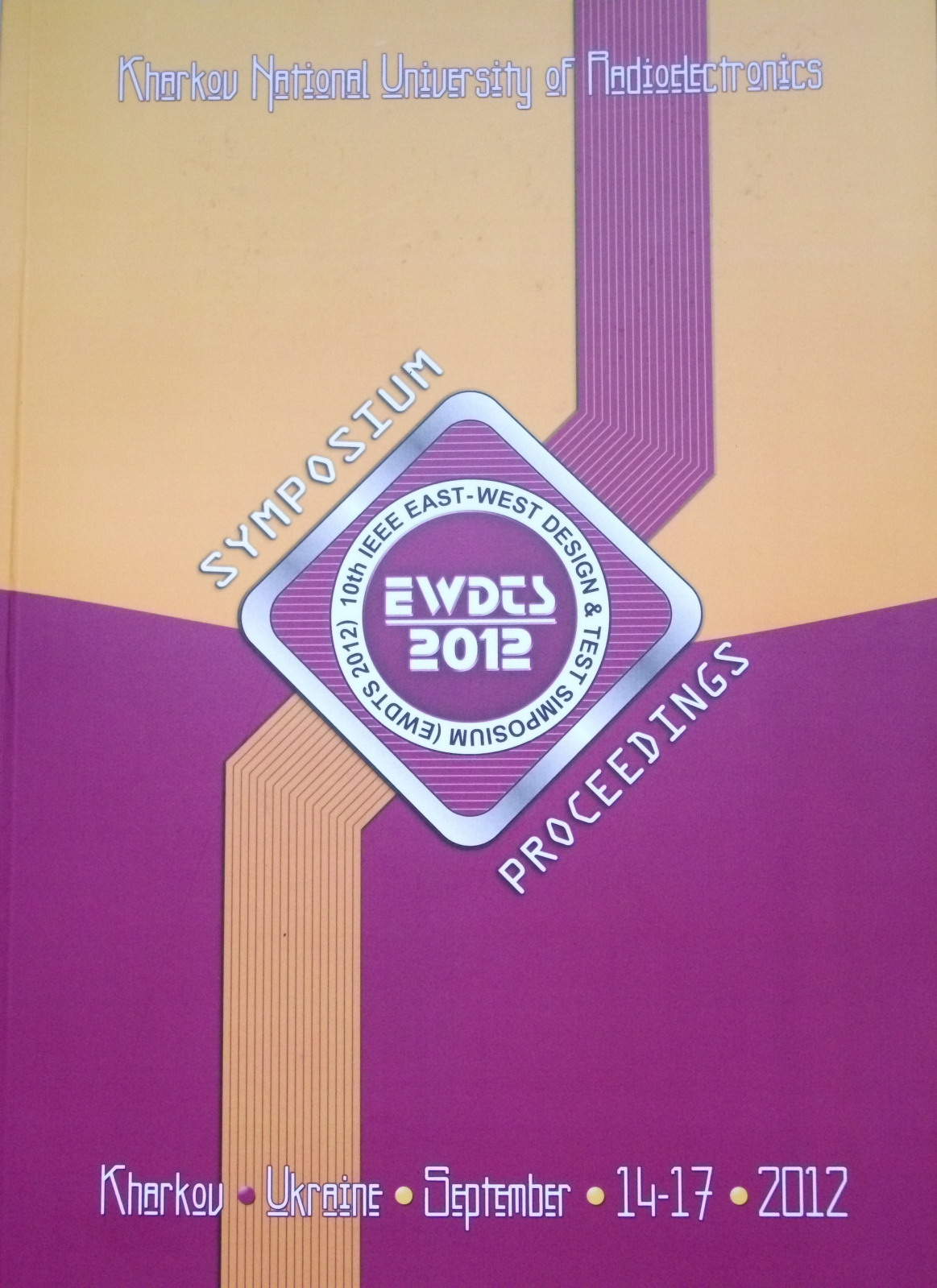• A
• A
• A
• ABC
• ABC
• ABC
• А
• А
• А
• А
• А
Regular version of the site

## Logi-Thermal Analysis of Digital Circuits Using Mixed-Signal Simulator Questa ADMS

P. 541-544.
Petrosyants K. O., Rjabov N.

CAD sybsystem for logi-thermal analysis of the digital circuits is presented. Logical circuits are described by VHDL language. Thermal regimes are calculated by electro-thermal analogy method. For the calculation of the thermal resistances and capacitances the thermal 3D simulator Overheat-IC based on semi-analytical solution of three-dimensional heat conduction equation is used. For simultaneous calculation of logical and thermal characteristics of IC analog and mixed-signal simulator Questa ADMS is used.

### In bookEdited by: S. Chumachenko, E. Litvinova. Kharkov: Kharkov national university of radioelectronics, 2012.